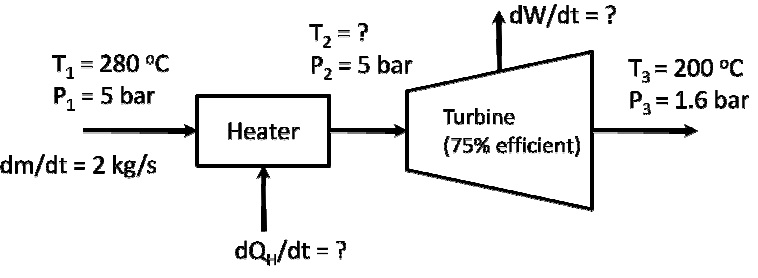I need to solve the material and energy balance

I need to solve the material and energy balance for the att.

Related Questions in Chemical Engineering

• Q :Rate of flow of nitrogen The feed to an

The feed to an ammonia synthesis reactor contain 25 mole% nitrogen and the balance hydrogen. The flow rate of the stream is 3000 kg/hr. Calculate the rate of flow of nitrogen into the reactor in kg/hr. (Hint: First calculate the average molecular weight of the mixture).

• Q :Problem on ideal gas law A stream of

A stream of steam at 1 bar and 400 K enters a compressor through a pipe with cross-sectional area of 0.1 m2 at a velocity of 5 m/s. The pressure of the outlet stream is at 5 bar, temperature is at 500 K, and velocity is 1 m/s. Assume the steam can be descri

• Q :Problem on Clausius-Clapeyron equation

The Clausius-Clapeyron equation gives us an expression for dP/dT. Now we will derive an analog to the Clausius-Clapeyron equation by obtaining an expression for dT/dµ when two phases are at equilibrium. For simplicity, let’s derive this fo

• Q :Problem on entropy A heater (heat

A heater (heat source temperature = 527 K) and turbine are connected in series as shown below:Q :Problem on empirical van Laar equation

At atmospheric pressure ethyl acetate and ethyl alcohol form an azeotropic mixture containing 53.9% mole of ethyl acetate and boiling at 71.8°C.

a) Estimate the values of A & B in the empirical van Laar equation

• Q :Thermodynamics Please can you look into

Please can you look into this assignment and let me know if its solve able.

• Q :Problem on weight fraction A gas

A gas contains 350 ppm of H2S in CO2 at 72°F and 1.53 atm pressure. If the gas is liquified, what is the weight fraction H2S?

• Q :Problem on frictional losses A stream

A stream of propane is throttled from 4.298 bar and 450 K to 0.22 bar. This process is irreversible due to frictional losses at the nozzle.

a. In words or equations, why are frictional losses irreversible?

Q :Estimating solubility of oxygen in water

The Henry's law constant for oxygen in water is as follows:

Temperature, °C                     0              &nbs

• Q :Hh to determine the temperature that

to determine the temperature that occurred in a fire in a warehouse, the arson investigator noticed that the relief valve on a methane storage tank had popped open at 3000 psig, the rated value. Before the fire started, the tank was presumably at ambient conditions and the gage read 1950 psig. If th